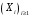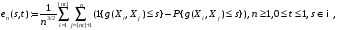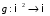# Convergence of the empirical two-sample -statistics with -mixing data

 bet 1/37 Sana 16.11.2020 Hajmi 380.03 Kb. #146511

(1 {g (Xi, Xj) 6 s} − P{g (Xi, Xj) 6 s})
CONVERGENCE OF THE EMPIRICAL TWO-SAMPLE -STATISTICS
WITH -MIXING DATA

HEROLD DEHLING, DAVIDE GIRAUDO AND OLIMJON SHARIPOV
Abstract. We consider the empirical two-sample -statistic with strictly -mixing strictly stationary data and inverstigate its convergence in Skorohod spaces. We then provide an application of such convergence.
1. INTRODUCTION AND MAIN RESULTS
Leta strictly stationary mixing sequence of real valued random variables. In this paper, we will study the asymptotic behavior of the empirical -statistic defined by(1,1)

wherekjR is a measurable function and for a real number x, [x] denotes the unique

integer k such that k 6 x < k + 1. The following is known:
In , the convergence of the 1-sample U-statistic defined by
1
 n3/2 X 16i6=j6n (1 {g (Xi, Xj) 6 s} - P {g (Xi, Xj) 6 s}) (1.2)

has been investigated.
In , the convergence of the 2-sample U-statistic of kernel h defined by
Tn (t) := 1
n3/2
[nt]
Xi
=1
nX
j=[nt]+1
h (Xi, Xj) (1.3)
has been investigated.
In this paper, we focus on the case of mixing sequences.
Let (Ω, F, P) be a probability space. The α-mixing and β-mixing coefficients between two
sub-σ-algebras A and B of F are defined defined respectively by
 α (A, B) = sup {|P (A ∩ B) - P (A) P (B)| , A ∈ A, B ∈ B} ; β (A, B) = 1 2 sup  IXi =1 JXj =1 |P (Ai ∩ Bj) - P (Ai) P (Bj)|  , (1.4) (1.5)  

CONVERGENCE OF THE EMPIRICAL TWO-SAMPLE STATISTICS WITH β-MIXING DATA
HEROLD DEHLING, DAVIDE GIRAUDO AND OLIMJON SHARIPOV
Abstract. We consider the empirical two-sample U-statistic with strictly β-mixing strictly stationary data and inverstigate its convergence in Skorohod spaces. We then provide an application of such convergence.

1. Introduction and main results

Let (Xi)i>1 a strictly stationary mixing sequence of real valued random variables. In this paper, we will study the asymptotic behavior of the empirical U-statistic defined by

 1 [nt] n en (s, t) := (1 {g (Xi, Xj) 6 s} − P{g (Xi, Xj) 6 s}) , n > 1, 0 6 t 6 1, s ∈ R, n3/2 i=1 nt X j=[X]+1

(1.1)
where g : R2 → R is a measurable function and for a real number x, [x] denotes the unique integer k such that k 6 x < k + 1. The following is known:

• In , the convergence of the 1-sample U-statistic defined by

1 X

(1.2)

n3/2
16i6=j6n
has been investigated.

• In , the convergence of the 2-sample U-statistic of kernel h defined by

 Tn (t) := 1 [nt] n h (Xi, Xj) (1.3) n 3/2 i=1 nt X j=[X]+1

has been investigated.
In this paper, we focus on the case of mixing sequences.
Let (Ω, F, P) be a probability space. The α-mixing and β-mixing coeﬃcients between two sub-σ-algebras A and B of F are defined defined respectively by
 α (A, B) = sup {|P(A ∩ B) − P(A) P(B)| , A ∈ A, B ∈ B} ; (1.4) 1 I J |P(Ai ∩ Bj) − P(Ai) P(Bj)| , β(A,B) = i=1 j=1 (1.5) 2 sup X X

Date: April 3, 2020.
Key words and phrases. U-statistics, empirical process .

1

k>1 kpα (k) converges.

• HEROLD DEHLING, DAVIDE GIRAUDO AND OLIMJON SHARIPOV

where the supremum runs over all the partitions (Ai)Ii=1 and (Bj)Jj=1 of Ω of elements of A and B respectively.

Given a sequence (Xi)i>1, we associate its sequences of α and β-mixing coeﬃcients by letting
 α ( k ) := sup α `, ∞ , (1.6) `>1 F1 F`+n β ( k ) := sup β `, ∞ , (1.7) `>1 F1 F`+n

where Fuv, 1 6 u 6 v 6 +∞ is the σ-algebra generated by the random variables Xi, u 6 i 6 v (u 6 i for v = +∞).
1.1. Convergence of the two-sample U-statistic in Skorohod spaces D ([−R, R] × [0, 1]).
Let us state one of the two main results of the paper.
Theorem 1.1. Let (Xi)iZ be a strictly stationary sequence. Let en be the two-sample U-statistics empirical process with kernel g : R × R R defined for n > 1, 0 6 t 6 1 and s R by
 1 [nt] n en (s, t) := (1 {g (Xi, Xj) 6 s} − P{g (Xi, Xj) 6 s}) . (1.8) n3/2 i=1 nt X j=[X]+1

Suppose that the following four conditions holds.
(A.1) For all u R, the random variable g (u, X1) has a density f1,u +∞.

(A.2) For all v R, the random variable g (X1, v) has a density f2,v
+∞.

P

(A.3) There exists a p > 2 such that

P

(A.4) The series k>1 kβ (k) converges.
Then for all R,
and supx,u∈R f1,u (x) <
and supx,v∈R f2,v (x) <

 en (s, t) → W (s, t) in distribution in D ([−R, R] × [0, 1]) , (1.9)

where (W (s, t) , s R, t [0, 1]) is a centered Gaussian process, with covariance given for 0 6 t 6 t0 6 1 and s, s0 R by the following formula:

 Cov (W (s, t) , W (s0, t0)) = t (1 − t) (1 − t0) C1,1 (s, s0) + t (1 − t0) (t0 − t) C2,1 (s, s0) + tt0 (1 − t0) C2,2 (s, s0) , (1.10) where for i, j = 1; 2 and s, s0 ∈ R, X (1.11) Ci,j (s, s0) = E[hi,s (X0) hj,s0 (Xk)] , k∈Z h1,s (u) = P{g (u, X1) 6 s} − P{g (X1, X2) 6 s} , (1.12) h2,s (v) = P{g (X1, v) 6 s} − P{g (X1, X2) 6 s} . (1.13)

Remark 1.2. We did not make a symmetry assumption on g. When g is symmetric, in the sense that g (u, v) = g (v, u) for all u and v ∈ R, the covariance of the limiting process W reads
 Cov (W (s, t) , W (s0, t0)) = t (1 − t0) (1 + 2t0 − 2t) C1,1 (s, s0) . (1.14)

3
In practical cases, the probability P{g (Xi, Xj) 6 s} is unknown, and we only have the values of 1 {g (Xi, Xj) 6 s}, 1 6 i < j 6 n, at our disposal. This leads to an analoguous result as Theorem 1.1, where the quantity P{g (Xi, Xj) 6 s} is replaced by its empirical estimator

n −1 P16i6n 1 {g (Xi, Xj) 6 s}.

2